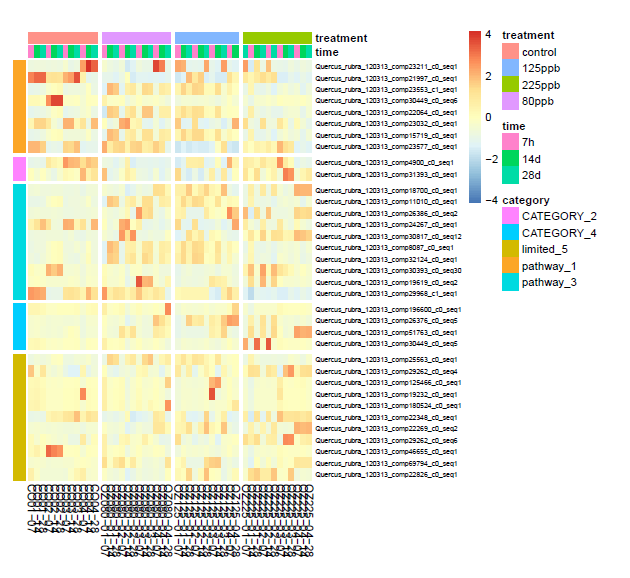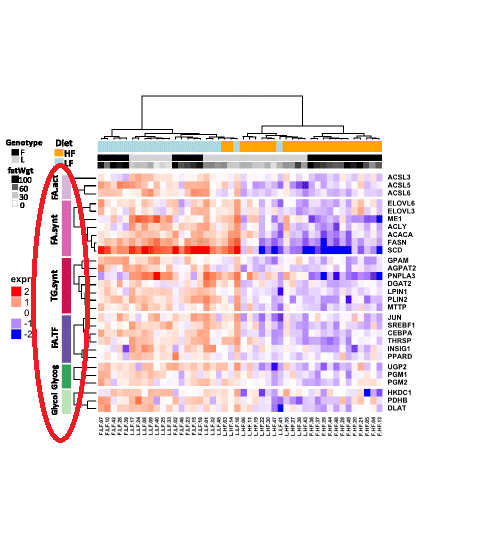Question: pheatmap: how to use legend information as row annotation?
0
n858250 wrote:

I am using below code in pheatmap to generate the first heatmap.

``````oz <- DESeq(oz)
rld <- rlog(oz)
topVarGenes <- head(order(rowVars(assay(rld)), decreasing = TRUE), 35)
mat  <- assay(rld)[topVarGenes, ]
mat  <- mat - rowMeans(mat)
anno_col <- as.data.frame(colData(rld)[, c("time","treatment")])
anno_row <- data.frame(category = c(rep("pathway_1", 8),
rep("CATEGORY_2", 2), rep("pathway_3", 10),
rep("CATEGORY_4", 4), rep("limited_5", 11)), stringsAsFactors = FALSE)
mixed_name <- cbind(mat[,0],anno_row)
pheatmap(mat, annotation_col = anno_col, annotation_row = mixed_name, scale = "column",
cluster_cols = F, cluster_rows= F, clustering_distance_rows = "correlation",
clustering_distance_cols = "correlation", clustering_method = "average",
gaps_row=c(8,10,20,24),
cellheight = 6, cellwidth = 10, border_color=NA, fontsize_row = 6)
``````Now, my question is that what should be added to the above code to have the "category" legend on the left of the heatmap and close to each category color, like the second figure that I have highlighted in red.annotation pheatmap R legend • 5.7k views
modified 2.3 years ago • written 2.3 years ago by n858250

Have you tried `annotation_name_row=T`? I think the legend position is an open issue.

Yes, I did. But nothing has changed.

1

You may want to take a look at the ComplexHeatmap package, with which you can definitely change the position of the legends and legend text.

I think you are right! The only way is switching to ComplexHeatmap package! Thank you!